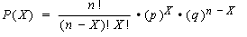# Binomial test / Exact Binomial Test

Share on

Binomial Theorem > Binomial test

## What is a binomial test?

The binomial test is used when an experiment has two possible outcomes (i.e. success/failure) and you have an idea about what the probability of success is. A binomial test is run to see if observed test results differ from what was expected.

Example: you theorize that 75% of physics students are male. You survey a random sample of 12 physics students and find that 7 are male. Do your results significantly differ from the expected results?

Solution: Use the binomial formula to find the probability of getting your results. The null hypothesis for this test is that your results do not differ significantly from what is expected.

Out of the two possible events, you want to solve for the event that gave you the least expected result. You expected 9 males (i.e. 75% of 12), but got 7, so for this example solve for 7 or fewer students.Note: you can find a step by step example of how to solve the equation here in the Binomial Formula article.

• p, the observed proportion, is 7/12, or .47.
• q, (1 – p) is 1 – .47.
• X is your expected number of successes (75% of 12 is 9).

Plugging those values into the formula and solving, you get:
0.158, which is the probability of 7 or fewer males out of 12. Doubling this (for a two tailed test), gives 0.315. These are your p-values. With very few exceptions, you’ll always use the doubled value.

As the p-value of 0.315 is large (I’m assuming a 5% alpha level here, which would mean p-values of less than 5% would be significant), you cannot reject the null hypothesis that the results are expected. In other words, 7 is not outside of the range of what you would expect.

If, on the other hand, you had run the test with 4 males (p=.333 and q=.666), the doubled p-value would have been .006, which means you would have rejected the null.

Another option: use an online calculator, like this one: VassarStats binomial calculator.

## Assumptions for the Binomial Test

1. Items are dichotomous (i.e. there are two of them) and nominal.
2. The sample size is significantly less than the population size.
3. The sample is a fair representation of the population.
4. Sample items are independent(one item has no bearing on the probability of another).
CITE THIS AS:
Stephanie Glen. "Binomial test / Exact Binomial Test" From StatisticsHowTo.com: Elementary Statistics for the rest of us! https://www.statisticshowto.com/binomial-test/
---------------------------------------------------------------------------Need help with a homework or test question? With Chegg Study, you can get step-by-step solutions to your questions from an expert in the field. Your first 30 minutes with a Chegg tutor is free!